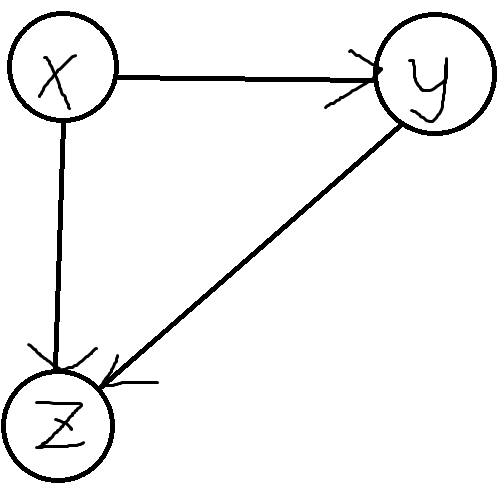## 题意：

If V=(A,B,C,D) and E=(AB,BC,CD,DA,AC), we call G as an “A-structure”.

## 思路：

“A-structure” 的实质就是两个三元环组成的，所以我们只要统计每条边参与了多少个三元环的组成，然后再用这个数套一下组合数就行啦。#include<bits/stdc++.h>
#define R register
#define fo(i, a, b) for (R int i = (a); i <= (b); ++i)
#define ll long long
#define N 600005
int n, m, cnt[N], head[N], nxt[N], to[N], tans, p[N], q[N];
ll ans;
std::vector <int> e[N];
struct edge{
int u, v;
}in[N];
int fl[N], tmp;
inline void add (int x, int y, int cnt)
{
to[cnt] = y;
}
int main()
{
while (scanf("%d %d", &n, &m) && n != 0 && m != 0)
{
memset(p, 0, sizeof(p));
ans = 0;
fo (i, 1, m)
{
scanf("%d %d", &in[i].u, &in[i].v);
cnt[in[i].u]++;
cnt[in[i].v]++;
}
fo (i, 1, m)
{
if (cnt[in[i].u] > cnt[in[i].v])
std::swap(in[i].u, in[i].v);
}
fo (i, 1, m)
{
tans = 0;
int u = in[i].u;
int v = in[i].v;
tmp++;
for (int j = head[u]; j != -1; j = nxt[j])
{
fl[to[j]] = tmp;
q[to[j]] = j;
}
for (int j = head[v]; j != -1; j = nxt[j])
if (fl[to[j]] == tmp)
{
p[j]++;
p[i]++;
p[q[to[j]]]++;
}
}
fo (i, 1, m)
ans = 1LL * ans + 1LL * p[i] * (p[i] - 1) / 2;
printf("%lld\n", ans);
}
return 0;
}